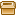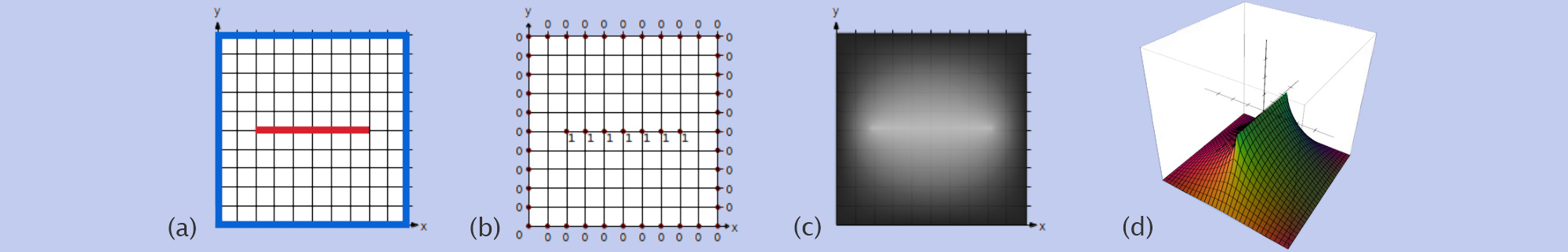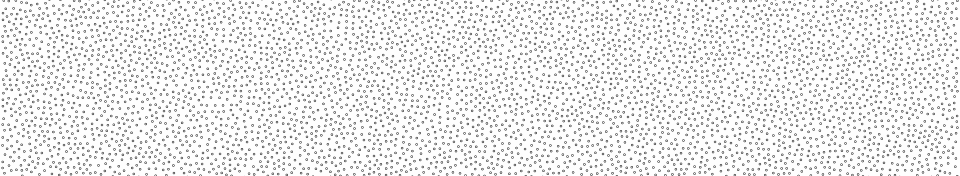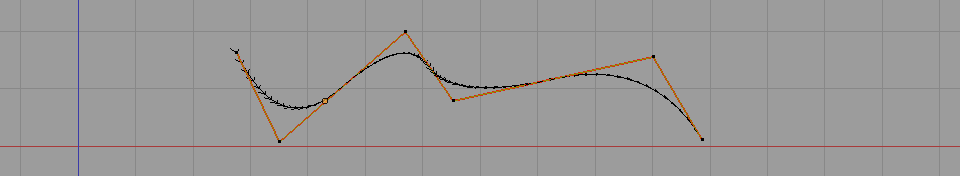# Find a tetrahedron circumcenter# Curvature of a parametric curve# Definition: Laplacian Matrix for triangle meshes# Diffusing / smoothing weight map over a triangular mesh.Showcasing simple procedures with C++ code to smooth / diffuse per vertex weights over a triangle mesh.

# Laplacian smoothing (C++ code to smooth a mesh)Dropping a procedure to compute the Laplacian smoothing of a 3D mesh (with cotangent weights).

# C++ code for cotangent weights over a triangular mesh# 2D biharmonic stencil a.k.a bilaplacian operatorDraft / notes / memo

# Voro++.0.4.5 with cmake for easy compilation under windowsDropping my code of thecmake version of voro++0.4.5 ]. It helped me to compile the voro++ library under windows with cmake.

# Harmonic function: definitions and propertiesThis is the second part of my tutorial series on bounded harmonic functions. For a quick introduction and examples of use of harmonic functions read the first part. In this part I define harmonic functions and their properties. This is the hard part with a lot of mathematics. But it's a mandatory step to understand how harmonic functions work. This will allow you to apply them in a broader context and understand many scientific papers relying on these. In addition this will be my entry point to introduce Finite Element Method in future posts. So hang on it's worth it!

# How to generate bounded harmonic weights on a regular grid# Source code for poisson disk sampling of a triangle mesh# Curvature of a triangle mesh, definition and computation.Defining and giving the formula to compute the curvature over a triangle mesh at some vertices.

# C++ code for spline curves# Compute Harmonic weights on a triangular meshHere I describe the discreet Laplace-Beltrami operator for a triangle mesh and how to derive the Laplacian matrix for that mesh. Then I provide [C++ code ] to compute harmonic weights over a triangular mesh by solving the Laplace equation.

• 1